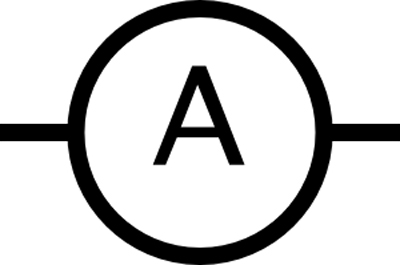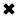# What is an Ampere? Basic Electrical Units#### The ampere is often called an

The ampere is a basic unit of electric current, often called an "amp". It is a unit of electrical constant current of one ampere. The ampere is one of several electrical charge units used to measure the electromagnetic force between straight parallel conductors carrying electric current. One ampere is equal to one coulomb of charge (or one newtons per metre) moving past a given it in one second. In terms of SI, the unit of charge, the coulomb is defined as the charge carried by one ampere during one second. Thus, current is a measure of the rate at which charge carriers flow. The standard unit is the ampere. This represents one coulomb (6,240,000,000,000,000,000) of charge carriers per second past a given point. An instrument called an ammeter is used to measure the flow in a circuit of parallel conductors of infinite length. The ammeter is inserted into the path of the current flow, or in series, to measure current. This means the circuit must be opened and the meter leads placed between the two open points.

Although the ammeter can measure electric flow in coulombs per second, it is calibrated or marked in amperes. For most practical applications, the term amperes is used instead of coulombs per second when referring to the amount of current flow. Note the use of the prefixes micro and milli to represent very small amounts of current and kilo and mega to represent very large amounts.

An ampere is a comparatively large amount of current. The abbreviation is A. Often, current which if maintained is specified in terms of milliamperes, abbreviated mA, where 1 mA = 0.001 A or a thousandth of an ampere. You will also sometimes hear of microamperes (uA), where 1 uA = 0.000001 A = 0. 001 mA, a millionth of an ampere. And it is increasingly common to hear about nanoamperes (nA), where 1 nA = 0.001 uA = 0.000000001 A (a billionth of an ampere). Rarely will you hear of kiloamperes (kA), where 1 kA = 1000 A.

A current of a few mUliamperes will give you a startling shock. About 50 mA will jolt you severely, and 100 mA can cause death if it flows through your chest cavity.

An ordinary 100-watt light bulb draws a rate of flow of about 1 A of current. An electric iron draws approximately 10 A; an entire household normally uses between 10 A and 50 A, depending on the size of the house and the kinds of appliances it has, and also on the time of day, week or year.

The amount of current that will flow in an electrical circuit depends on the voltage, and also on the resistance. There are some circuits in which extremely large currents, say 1000 A, flow; this might happen through a metal bar placed directly at the output of a massive electric generator. The resistance is extremely low in this case, and the gen­erator is capable of driving huge amounts of charge. In some semiconductor electronic devices, such as microcomputers, a few nanoamperes will suffice for many complicated processes. Some electronic clocks draw so little current that their batteries last as long as they would if left on the shelf without being put to any use at all.

##### Basic Electricity Handbook, Vol. 1• FULLY ILLUSTRATED
• GREAT FOR SCHOOL PROJECTS
• GREAT PRICE: \$5.99
• ...

This 100+ page e-book is a great guide for those who have a basic interest in the field of electricity. This well-illustrated e-book, coupled with some basic knowledge of electricity, will give you a broad theoretical background in this fundamental subject.

CONTENTS
• FUNDAMENTALS OF ELECTRICITY
• HOW DOES ELECTRICITY WORK?
• HOW IS ELECTRICITY GENERATED?
• WHERE DOES ELECTRICITY COME FROM?
• BASIC HOME WIRING
• ELECTRICITY FOR STUDENTS
• ENERGY SAVING TIPS

##### TRAINING EF COURSES

Content Community Connection
Top